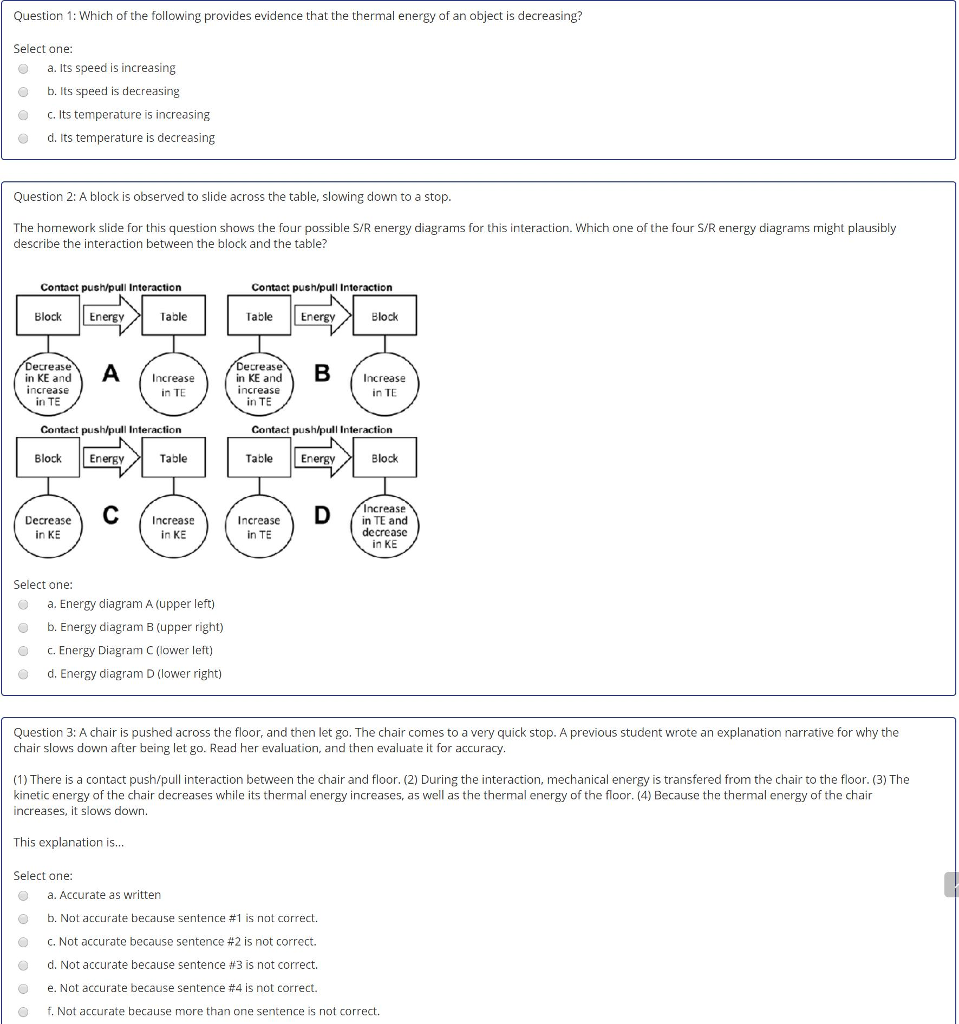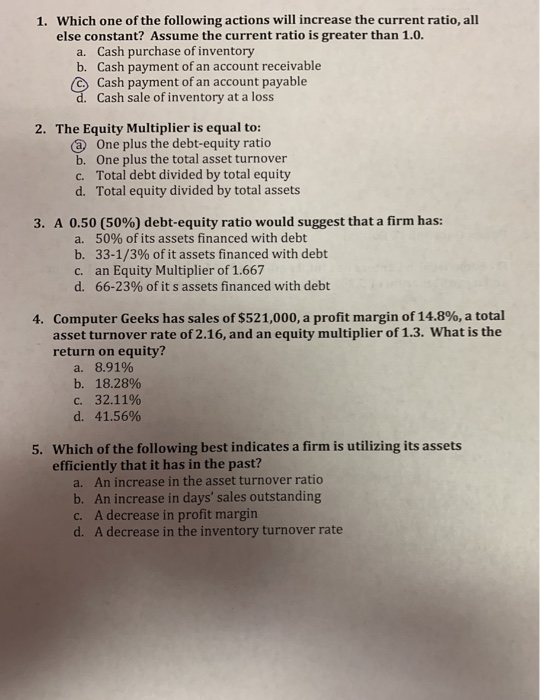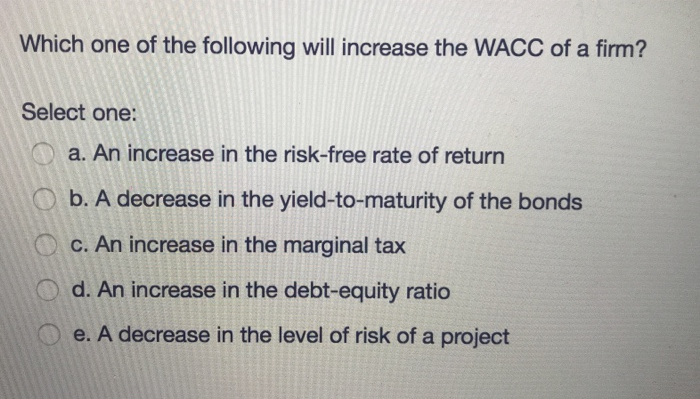# Which of the following actions will increase the energy of an object? Select one: a. Lifting...

Which of the following actions will increase the energy of an object? Select one: a. Lifting it from the ground and placing it on a shelf b. Increasing its velocity by throwing it across the room c. Stretching or compressing the object d. All of these actions will increase the energy of an object

It's answer is option number d.

All of these actions will increase the energy of an object.

Lifting will provide potential energy,

Throwing will give kinetic energy,

Stretching and compressing will give elastic potential energy.

#### Earn Coin

Coins can be redeemed for fabulous gifts.

Similar Homework Help Questions
• ### Question 1: Which of the following provides evidence that the thermal energy of an object is...Question 1: Which of the following provides evidence that the thermal energy of an object is decreasing? Select one: a. Its speed is increasing b. ts speed is decreasing C. Its temperature is increasing d. Its temperature is decreasing Question 2: A block is observed to slide across the table, slowing down to a stop The homework slide for this question shows the four possible S/R energy diagrams for this interaction. Which one of the four S/R energy diagrams might...

• ### Which of the following will increase revenue per available room? Select one: a. Decrease of the...

Which of the following will increase revenue per available room? Select one: a. Decrease of the ADR when demand for rooms is low b. Increase of the ADR when demand for rooms is high c. Increasing the hotel rates in a low season d. Decrease of the ADR when demand for rooms is stable

• ### Which would increase the internal energy of your body (the system)? A. exercising by lifting weights...

Which would increase the internal energy of your body (the system)? A. exercising by lifting weights B. going outside on a cold day C. drinking a cup of hot chocolate D. taking a cold bath E. None of these

• ### Which of the following Fed actions will increase bank lending? Select one or more answers from...

Which of the following Fed actions will increase bank lending? Select one or more answers from the choices shown   The Fed lowers the discount rate from 4 percent to 2 percent The Fed raises the reserve ratio from 10 percent to 11 percent  The Fed raises the discount rate from 5 percent to 6 percent The Fed sells bonds to commercial banks

• ### Which of the following actions will increase the equilibrium concentration of a gas in water?      ...

Which of the following actions will increase the equilibrium concentration of a gas in water?       1. increasing the temperature of the water       2. increasing the volume water       3. increasing the pressure of the gas above the liquid a) 1 only             b) 2 only             c) 3 only             d) 1 and 3           3) 1, 2, and 3

• ### Which of the following Fed actions will increase bank lending? You may select more than one...

Which of the following Fed actions will increase bank lending? You may select more than one answer. The Fed raises the discount rate from 5 percent to 6 percent. The Fed raises the reserve ratio from 10 percent to 11 percent. The Fed lowers the discount rate from 4 percent to 2 percent. The Fed buys \$400 million worth of Treasury bonds from commercial banks.

• ### 1. Two objects collide and stick together. Kinetic energy Select one: a. unable to determine with...

1. Two objects collide and stick together. Kinetic energy Select one: a. unable to determine with the information. b. Kinetic energy is conserved. c. Kinetic energy is not conserved. d. is conserved only if the environment is frictionless. 2. A ball of mass 0.10 kg is dropped from a height of 12 m. Its momentum when it strikes the ground is Select one: a. 2.4 kg·m/s. b. 1.8 kg·m/s. c. 1.5 kg·m/s. d. 4.8 kg·m/s. 3. If you double the...

• ### 1. Which one of the following actions will increase the current ratio, all else constant? Assume...1. Which one of the following actions will increase the current ratio, all else constant? Assume the current ratio is greater than 1.0. a. Cash purchase of inventory b. Cash payment of an account receivable C Cash payment of an account payable d. Cash sale of inventory at a loss 2. The Equity Multiplier is equal to: @ One plus the debt-equity ratio b. One plus the total asset turnover C. Total debt divided by total equity d. Total equity...

• ### If the velocity of an object is doubled and its mass is halved, which of the...

If the velocity of an object is doubled and its mass is halved, which of the following statements is true about the object? Select one: a. Its momentum is halved; its kinetic energy doubles. b. Its momentum stays the same; its kinetic energy doubles. c. Its momentum is halved; its kinetic energy quadruples. d. Its momentum is halved; its kinetic energy is also halved.

• ### Which one of the following will increase the WACC of a firm? Select one: a. An...Which one of the following will increase the WACC of a firm? Select one: a. An increase in the risk-free rate of return b. A decrease in the yield-to-maturity of the bonds C. An increase in the marginal tax O d. An increase in the debt-equity ratio Oe. A decrease in the level of risk of a project